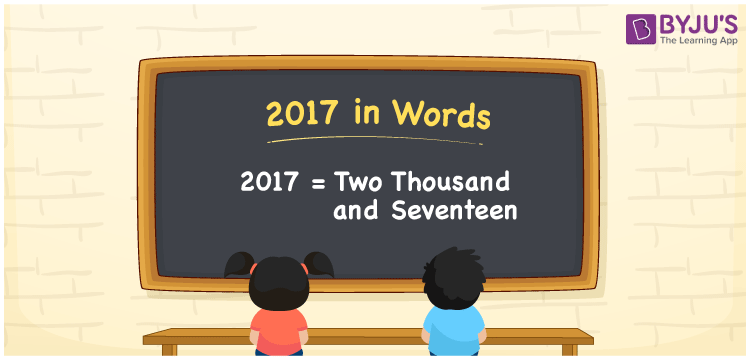# 2017 in Words

2017 in words is written as Two thousand seventeen. In both the International System of Numerals and the Indian System of Numerals, 2017 is written as Two thousand seventeen. The number 2017 is a Cardinal Number as it could represent some quantity.

 2017 in Words Two thousand seventeen Two thousand seventeen in Number 2017

## 2017 in English Words

2017 in English words is read as “Two thousand seventeen”.## How to Write 2017 in Words?

To write 2017 in words, we shall use the place value chart. In the place value chart, put 2 in the thousands, 0 in the hundreds, 1 in the tens, and 7 in the ones, respectively. Let us make a place value chart to write the number 2017 in words.

 Thousands Hundreds Tens Ones 2 0 1 7

Thus, we can write the expanded form as

2 × Thousand + 0 × Hundred + 1 × Ten + 7 × One

= 2 × 1000 + 0 × 100 + 1 × 10 + 7 × 1

= 2000 + 0 + 10 + 7

= 2017

= Two thousand seventeen.

2017 is a natural number, the successor of 2016 and the predecessor of 2018.

2017 in words – Two thousand seventeen

• Is 2017 an odd number? – Yes
• Is 2017 an even number? – No
• Is 2017 a perfect square number? – No
• Is 2017 a perfect cube number? – No
• Is 2017 a prime number? – Yes
• Is 2017 a composite number? – No

## Frequently Asked Questions on 2017 in Words

Q1

### How to write 2017 in words?

2017 in words is written as Two thousand seventeen.
Q2

### How to write 2017 in the International and Indian System of Numerals?

In both, the system of numerals, 2017 in words, is written as Two thousand seventeen.
Q3

### How to write 2017 in a place value chart?

In the place value chart, write 2 in the thousands, 0 in the hundreds, 1 in the tens, and 7 in the ones, respectively.